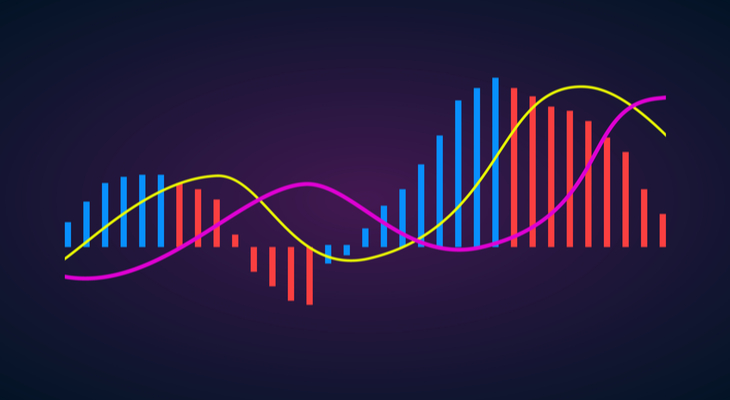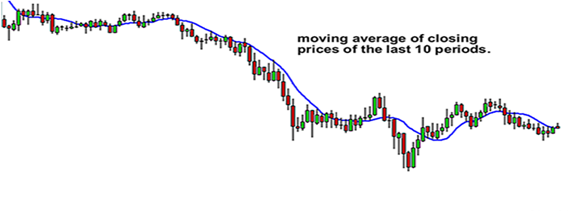## How To Use Moving Averages To Buy StocksWhen it comes to trading stocks, it is important that traders know how to use indicators to enter and exit trades.

One key chart indicator, which day traders use to determine where stability lies, is the Moving Average (MA).

Moving averages are popular among day traders, investors, and technical analysts around the globe.

These people use moving averages on charts to help determine direction, strength, trend, and to find entry and exit points.

This article discusses what moving averages are, the different types, why they are important, and how day traders can use them to enter trades.

### What are Moving Averages?

A moving average (MA) is a technical indicator that combines price points of a given instrument, such as a stock, over a specified time frame, and divides by the number of prices points to give you a single trend line.

Moving averages can be calculated for any sequential data set, including trading volume, highest and lowest prices, opening and closing prices, or any other indicators.

The following diagram shows how a moving average would look like on a chart:As previously mentioned, MAs are popular among traders and technical analysts.

They rely on them to filter out the ‘noise’ and calculate the average value of a stock price over a specific period.

Moving averages can also help to determine the direction of the current trend while lessening the impact of random price spikes.

They are known as ‘moving’ because the introduction of new price points will replace old price points and ‘move’ the line on the chart.

### Types of Moving Averages

There are four different types of moving averages, which we have explained below:

1. Simple Moving Average (SMA)

Simple moving average, also known as an arithmetic moving average, is calculated by summing up a selected range of prices, usually closing prices, and then dividing the sum by the number of periods in that range.